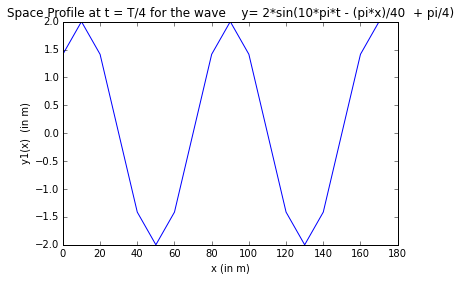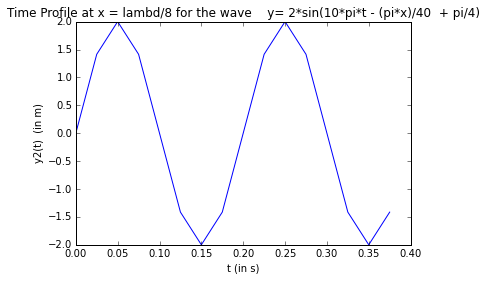Chapter 2 - What is Light¶

Example 2 - pg 32¶

In :
#plot the required space and time profiles
%matplotlib inline
# wave y= 2*sin(10*pi*t - (pi*x)/40  + pi/4)
# (a) Plot the space profile at t= T/4
# Comapring the given Equation with  y= A*sin(omega*t - k*x + phi)
#given
import math,matplotlib,numpy
from matplotlib import pyplot
omega = 10*math.pi ; #Angular frequency in rad/s
k= math.pi/40. ; # Wave number in rad/m
T= 1/5. ; # 2*pi/T = 10*pi , so Time period is 1/5 s
lambd = 80.; # Wavelength in m ,  2*pi/lambd = pi/40 , so lambd = 80
#calculations
t1= T/4; #time period in s
x1= 0.;#  in m
print"The Space profile of a wave y= 2*sin(10*pi*t - (pi*x)/40 + pi/4) when  t= T/4\n\n"
print"\tx (in m) \t y1(x)  (in m)\n"
while x1<180:
y1= 2*math.sin((omega*t1)-(k*x1)+ (math.pi/4));
print"\t",x1,"\t\t",y1,"\n"
x1 = x1+10;

#Now, we will plot the space profile from the values obtained for y1 for each value of x1
x_1 = numpy.array([0,10.,20.,30.,40.,50.,60.,70.,80.,90.,100.,110.,120.,130.,140.,150.,160.,170.]);
y_1 = numpy.array([1.414214,2.000000,1.414214,0.000000,-1.414214,-2.000000,-1.414214,-0.000000,1.414214,2.000000,1.414214,0.000000,-1.414214,-2.000000,-1.414214,-0.000000,1.414214,2.000000]);
# axis centered at (0,0)
pyplot.plot(x_1,y_1);
pyplot.title("Space Profile at t = T/4 for the wave    y= 2*sin(10*pi*t - (pi*x)/40  + pi/4)")
pyplot.xlabel("x (in m)")
pyplot.ylabel("y1(x)  (in m)")
pyplot.show()
#(b)
x2= lambd/8.; #in m
t2=0; # time period in s
print"The time profile of a wave y= 2*sin(10*pi*t - (pi*x)/40 + pi/4) when  x= lambd/8\n\n"
print"\t t(in s)     \t     y2(t) (in m)\n\n"
while t2<0.4:
y2=2*math.sin((omega*t2)-(k*x2)+ (math.pi/4));
print "\t",x2,"\t\t",y2,"\n"
t2=t2+0.025;

#Now,we will plot the time profile from the values obtained for y2 ,for each value of t2
x_2=numpy.array([0,0.025,0.05,0.075,0.1,0.125,0.15,0.175,0.2,0.22500,0.250000,0.27500,0.30000,0.325000,0.350000,0.37500])
y_2=numpy.array([0.000000,1.414214,2.000000,1.414214,0.000000,-1.414214,-2.000000,-1.414214,-0.000000,1.414214,2.000000,1.414214,0.000000,-1.414214,-2.000000,-1.414214])
# axis centered at (0,0)
pyplot.plot(x_2,y_2);
pyplot.title("Time Profile at x = lambd/8 for the wave    y= 2*sin(10*pi*t - (pi*x)/40  + pi/4)")
pyplot.xlabel("t (in s)")
pyplot.ylabel("y2(t)  (in m)")
pyplot.show()
The Space profile of a wave y= 2*sin(10*pi*t - (pi*x)/40 + pi/4) when  t= T/4

x (in m) 	 y1(x)  (in m)

0.0 		1.41421356237

10.0 		2.0

20.0 		1.41421356237

30.0 		0.0

40.0 		-1.41421356237

50.0 		-2.0

60.0 		-1.41421356237

70.0 		-2.44929359829e-16

80.0 		1.41421356237

90.0 		2.0

100.0 		1.41421356237

110.0 		2.26621555906e-15

120.0 		-1.41421356237

130.0 		-2.0

140.0 		-1.41421356237

150.0 		-7.34788079488e-16

160.0 		1.41421356237

170.0 		2.0The time profile of a wave y= 2*sin(10*pi*t - (pi*x)/40 + pi/4) when  x= lambd/8

t(in s)     	     y2(t) (in m)

10.0 		0.0

10.0 		1.41421356237

10.0 		2.0

10.0 		1.41421356237

10.0 		2.44929359829e-16

10.0 		-1.41421356237

10.0 		-2.0

10.0 		-1.41421356237

10.0 		-2.26621555906e-15

10.0 		1.41421356237

10.0 		2.0

10.0 		1.41421356237

10.0 		7.34788079488e-16

10.0 		-1.41421356237

10.0 		-2.0

10.0 		-1.41421356237Example 3 - pg 33¶

In :
#calculate the velocity of wave and Intensity
#given
import math
from math import sin,asin
#Let us consider, wave function  y = A*sin(omega*t - K*x + phi)
A= 0.02;# Amplitude in m
lambd = 6; # Wavelength (lambd) = Crest Distance = 6 m
T= 2.;# Time period is s
#calculations and results
nu =  1/T; # Frequency in Hz
omega = 2*math.pi*nu ; #Angular Frequency in rad/s
k = 2*math.pi/lambd; #wave number in rad/m
#from Space profile, when x=1.5 m, t= 0
y = 0.02; #in m
x=1.5;#in m
t= 0; # in s
phi = (asin(y/A) +(k*x) - (omega*t)); # Initial phase in radians
print" Wave parameters from the space profile and time profile\n"
print" (1)Amplitude is (m) = ",A
print" (2)Wavelength  is (m) = ",lambd
print" (3)Time period is (s) = ",T
print" (4)Frequency is (Hz) = ",nu
print" (5)Angular Frequency is (rad/s) =",round(omega,2)
print" (6)Wave number is (rad/m) = ",round(k,2)
print" (7)Initial phase is (radians) = ",round(phi,2)
# y(x,t=0) : -0.02 = 0.02*sin(0-(pi*x)/3 + pi)
#Thus  (-pi*x)/3 + pi = -pi/2,-5*pi/2, giving x= 9/2 m,21/2m
V= omega/k; # Velocity of wave in m/s
# I is proportional to A**2
I = A**2; # Intensity in m**2 (Proportional)
print" (8)The velocity of wave is (m/s) = ",V
print" (9)Intensity is proportional to :",I*10**4,"x 10^-4 m^2."
Wave parameters from the space profile and time profile

(1)Amplitude is (m) =  0.02
(2)Wavelength  is (m) =  6
(3)Time period is (s) =  2.0
(4)Frequency is (Hz) =  0.5
(5)Angular Frequency is (rad/s) = 3.14
(6)Wave number is (rad/m) =  1.05
(7)Initial phase is (radians) =  3.14
(8)The velocity of wave is (m/s) =  3.0
(9)Intensity is proportional to : 4.0 x 10^-4 m^2.

Example 6 - pg 39¶

In :
#calculate the parameters for light and sound waves
#(a)Tunning fork
#given
import math
nu= 440.; # Frequency in Hz
V=340.; # velocity of sound in air in m/s
#calculations
lambd= V/nu ;# Wavelength of sound wave in m
k= 2*math.pi/lambd; # Wave number in m
#(b) Red Light
nu1 = 5.*10**14;# Frequency of Red light in Hz
V1 = 3.*10**8;#Velocity of light in m/s
lambd1= V1/nu1; #Wavelength of light wave in m
k1= 2*math.pi/lambd1; # Wave number in m
#results
print "For Sound wave :"
print "Frequency: (Hz) = ",nu
print "Velocity: (m/s) = ",V
print "Wavelegth: (m) = ",round(lambd,3)
print "Wave number : (m) =",round(k,2)
print "Wave Equation for Sound wave: y =  A*sin((",round(k,2),"*x)-(",round((2*math.pi*nu),2),"*t)) "
print "For Light wave :"
print "Frequency: ",nu1*10**-14," x 10^14 Hz"
print "Velocity:",V1*10**-8," x 10^8 m/s "
print "Wavelegth:",lambd1*10**7," x 10^-7 m"
print "Wave number : ",round(k1*10**-7,2),"10^7 m"
print "Wave Equation for Sound wave: y =  A*sin((",round(k1*10**-7,2),"*10^7*x)-(",round(2*math.pi*nu1*10**-15,2),"*10^15*t))"
For Sound wave :
Frequency: (Hz) =  440.0
Velocity: (m/s) =  340.0
Wavelegth: (m) =  0.773
Wave number : (m) = 8.13
Wave Equation for Sound wave: y =  A*sin(( 8.13 *x)-( 2764.6 *t))
For Light wave :
Frequency:  5.0  x 10^14 Hz
Velocity: 3.0  x 10^8 m/s
Wavelegth: 6.0  x 10^-7 m
Wave number :  1.05 10^7 m
Wave Equation for Sound wave: y =  A*sin(( 1.05 *10^7*x)-( 3.14 *10^15*t))